﻿ 杨义军 博士-人机交互与虚拟现实研究中心杨义军 博士

 Yi-Jun Yang(杨义军)（更多内容） Correspondence: School of Computer Science and Technology, Software Park Campus, Shandong University, No.1500, Shunhua Road, Jinan, Shandong Province, P.R. China Zip Code: 250101 Email: yangyijuny AT gmail.com or yyj AT sdu.edu.cn URL: http://vr.sdu.edu.cn/~yyjBio Sketch I am now an associate professor in School of Computer Science and Technology of Shandong University. In 07/2008, I received my Ph.D degree in the Department of Computer Science and Technology at Tsinghua University, China. I received my B.Sc. and M. Sc in Computer Science and Technology from Shandong University in 2000 and 2003, respectively. Research Interests Constrained Surface Mapping Extremal Quasiconformal Mapping Surface Registration and Analysis             Freeform Surface Parameterization
 Publications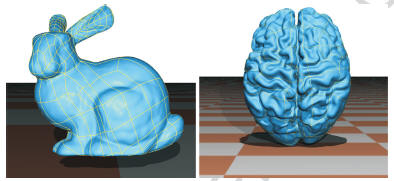Y.J. Yang, W. Zeng, Xiang-Xu Meng. Conformal freeform surfaces. Computer-Aided Design, 2016, 81:48-60.Abstract: The conformality of freeform surfaces highly affects their rendering and tessellation results. To improve the conformality of freeform surfaces, a novel freeform surface representation named hierarchical freeform surfaces is presented in this paper. The conformality energy of hierarchical freeform surfaces is first formulated and its numerical approximation is then constructed using the composite Simpson’s rule. By constructing the parameterization of the initial freeform transformation using the Ricci flow method, the optimal freeform transformation is obtained by the Levenberg–Marquardt method, which is further interleaved with the freeform refinement procedure to generate a hierarchical freeform surface with bounded conformality deviations. Examples are given to show the performance of our algorithm for rendering and tessellation applications.W. Zeng, Y.J. Yang and Muhammad Razib. Graph-Constrained Surface Registration Based on Tutte Embedding. The 7th International Workshop on Biomedical Image Registration, 2016.Abstract: This work presents an efficient method to compute the registration between surfaces with consistent graph constraints based on Tutte graph embedding. Most natural objects have consistent anatomical structures, extracted as isomorphic feature graphs. For genus zero surfaces with ≥ 1 boundaries, the graphs are planar and usually 3-connected. By using Tutte embedding, each feature graph is embedded as a convex subdivision of a planar convex domain. Using the convex subdivision as constraint, surfaces are mapped onto convex subdivision domains and the registration is then computed over them. The computation is based on con-strained harmonic maps to minimize the stretching energy, where curvy graph constraints become linear ones. This method is theoretically rigorous. The algorithm solves sparse linear systems and is computationally efficient and robust. The resulting mappings are proved to be unique and diffeomorphic. Experiments on various facial surface data demonstrate its efficiency and practicality.Y.J. Yang, W. Zeng, Tian-Qi Song.Optimizing Conformality of NURBS Surfaces by General Bilinear Transformations. Computer-Aided Design,2015,46(6):12–25.SCI,EIAbstract: The conformality of NURBS surfaces greatly affects the results of rendering and tessellation applications. To improve the conformality of NURBS surfaces, an optimization algorithm using general bilinear transformations is presented in this paper. The conformality energy is first formulated and its numerical approximation is then constructed using the composite simpson’s rule. The initial general bilinear transformation is obtained by approximating the conformal mapping of its 3D discretized mesh using a least square method, which is further optimized by the Levenberg–Marquardt method. Examples are given to show the performance of our algorithm for rendering and tessellation applications.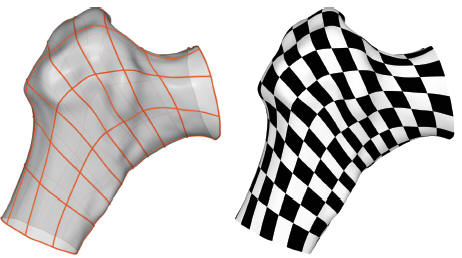Y.J. Yang, W. Zeng. Optimizing Equiareality of NURBS Surfaces using Composite Mobius Transformations. Journal of Computational and Applied Mathematics(CAM),2015,279:1-12.SCI,EIAbstract: The equiareality of NURBS surfaces greatly affects the results of visualization and tessellation applications, especially when dealing with extruding and intruding shapes. To obtain more equiareal parameterizations of NURBS surfaces than the Mobius based method, an optimization algorithm is presented in this paper based on the more flexible composite reparameterization functions. For fixed knots, the optimal composite reparameterization can be obtained using the Levenberg-Marquardt method. For a given tolerance, a uniform subdivision scheme is interleaved with the optimization procedure and this process finishes until the change of the equiareal deviation energy is less than the given tolerance. Examples are given to show the performance of our algorithm for visualization and tessellation applications.W. Zeng, Y.J. Yang. Colon Flattening by Landmark-Driven Optimal Quasiconformal Mapping. MICCAI 2014.Abstract: In virtual colonoscopy, colon conformal flattening plays an important role, which unfolds the colon wall surface to a rectangle planar image and preserves local shapes by conformal mapping, so that the cancerous polyps and other abnormalities can be easily and thoroughly recognized and visualized without missing hidden areas. In such maps, the anatomical landmarks (taeniae coli, flexures, and haustral folds) are naturally mapped to convoluted curves on 2D domain, which poses difficulty for comparing shapes from geometric feature details. Understanding the nature of landmark curves to the whole surface structure is meaningful but it remains challenging and open. In this work, we present a novel and effective colon flattening method based on quasiconformal mapping, which straightens the main anatomical landmark curves with least conformality (angle) distortion. It provides a canonical and straightforward view of the long, convoluted and folded tubular colon surface. The computation is based on the holomorphic 1-form method with landmark straightening constraints and the quasiconformal optimization, and has linear time complexity due to the linearity of 1-forms in each iteration. Experiments on various colon data demonstrate the efficiency and efficacy of our algorithm and its practicability for polyp detection and findings visualization; furthermore, the result reveals the geometric characteristics of anatomical landmarks on the colon surfaces.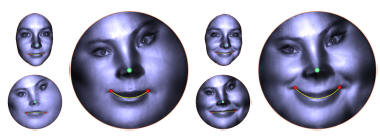W. Zeng, Y.J. Yang.  Surface Matching and Registration by Landmark Curve-Driven Canonical Quasiconformal Mapping. ECCV 2014.Abstract: This work presents a novel surface matching and registration method based on the landmark curve-driven canonical surface quasiconformal mapping, where an open genus zero surface decorated with landmark curves is mapped to a canonical domain with horizontal or vertical straight segments and the local shapes are preserved as much as possible. The key idea of the canonical mapping is to minimize the harmonic energy with the landmark curve straightening constraints to generate a quasi-holomorphic 1-form which is zero in one parameter along landmark and results in a quasiconformal mapping. The mapping exists and is unique and intrinsic to surface and landmark geometry. The novel shape representation provides a conformal invariant shape signature; we use this as Teichm¨uller coordinates to construct a subspace of the Teichm¨uller space which considers geometry feature details and therefore increases the discriminative ability for matching. Furthermore, we present a novel and efficient registration method for surfaces with landmark curve constraints by computing an optimal mapping over the canonical domains with straight segments, where the curve constraints become linear forms. Due to the linearity of 1-form and harmonic map, the algorithms are easy to compute, efficient and practical. Experiments on human face and brain surfaces demonstrate the efficiency and efficacy and shows the potential for broader shape analysis applications.Y.J. Yang,  W. Zeng, J.F. Chen. Equiareal parameterizations of NURBS surfaces. Graphical Models (GMOD),2014,76(1):43-55.  (SCI,EI, impact factor: 0.697) Abstract: The equiareality of freeform surfaces greatly affects the results of visualization and tessellation applications, especially when dealing with extruding and intruding shapes. To improve the equiareality of given freeform surfaces, an optimization algorithm using the Mobius transformations is presented in this paper. The optimal Mobius transformation is obtained by computing the intersection  of two planar algebraic curves, whose coefficients are computed explicitly for Bezier and B-spline surfaces, while numerically for NURBS surfaces. Examples are given to show the performance of our algorithm for visualization and tessellation applications.C.L. Yang, W.Z. Wang, Y.J. Yang, L. Lu, Z.J. Zhu, B.H. Zhu, W. Zeng. Weak visibility polygons of NURBS curves inside simple polygons. Journal of Computational and Applied Mathematics,2014,256(1-15). (SCI:EI, impact factor 0.989)Abstract: Visibility computation plays an important role in applications such as architectural design, art gallery patrolling and virtual worlds. In this paper, we present an algorithm to compute the weak visibility polygons (WVP) of Non Uniform Rational B-spline (NURBS) curves inside simple polygons. The NURBS curve is first subdivided into triangular curves. We then compute the WVP of each triangular curve by shearing that of its triangle hull. Finally, all triangular curves’ WVPs are merged together to obtain the WVP of the NURBS curve. Analysis and examples are given to show the performance of our algorithm.Y.J. Yang, , W. Zeng, C.L. Yang, B.L. Deng, X.X. Meng,S.S. lyengar. An algorithm to improve parameterizations of rational Bezier surfaces using rational bilinear reparameterization. Computer-Aided Design,2013,45(3):628–638.(SCI: 102GY; EI:20130215873983,impact factor 1.264)Abstract: The representation of rational Bezier surfaces greatly affects the results of rendering and tessellation applications. The uniformity and orthogonality of iso-parameter curves are two key properties of the optimal parameterization. The only rational Bezier surfaces with uniform iso-parameter curves are bilinear surfaces, while the control points of the rational Bezier surfaces with orthogonal iso-parameter curves satisfy a symmetrical condition. Moreover, the only rational Bezier surfaces with uniform and orthogonal iso-parameter curves are rectangles. To improve the uniformity and orthogonality of iso-parameter curves for general Bezier surfaces, an optimization algorithm using the quadratic transformations is presented, which can produce a better parameterization with the cost of degree elevation. Examples are given to show the performance of our algorithm for rendering and tessellation applications.Y.J. Yang, W. Zeng, C.L. Yang, X.X. Meng, J.H. Yong, B.L. Deng. G1 continuous approximate curves on NURBS surfaces. Computer-Aided Design,2012,44(9):824–834. (SCI: 966SW; EI: 20122315101838,  impact factor 1.264) Abstract: Curves on surfaces play an important role in computer aided geometric design. In this paper, we present a parabola approximation method based on the cubic reparameterization of rational Bezier surfaces, which generates G1 continuous approximate curves lying completely on the surfaces by using iso-parameter curves of the reparameterized surfaces. The Hausdorff distance between the approximate curve and the exact curve is controlled under the user-specified tolerance. Examples are given to show the performance of our algorithm.Y.L. Yang, Y.J. Yang, M. Niloy, H. Pottmann. Shape space exploration of constrained meshes. ACM transaction on Graphics (TOG),2011,30(6). (SCI: 856YP; EI: 20114914575468, impact factor 3.489). SIGGRAPH ASIA 2011.Abstract: We present a general computational framework to locally characterize any shape space of meshes implicitly prescribed by a collection of non-linear constraints. We computationally access such manifolds, typically of high dimension and co-dimension, through first and second order approximants, namely tangent spaces and quadratically parameterized osculant surfaces. Exploration and navigation of desirable subspaces of the shape space with regard to application specific quality measures are enabled using approximants that are intrinsic to the underlying manifold and directly computable in the parameter space of the osculant surface. We demonstrate our framework on shape spaces of planar quad (PQ) meshes, where each mesh face is constrained to be (nearly) planar, and circular meshes, where each face has a circumcircle. We evaluate our framework for navigation and design exploration on a variety of inputs, while keeping context specific properties such as fairness, proximity to a reference surface, etc.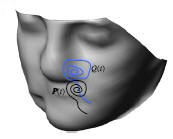H. C. Song, J.H. Yong, Y. J. Yang, X.M. Liu. Algorithm for orthogonal projection of parametric curves on B-spline surfaces. Computer-Aided Design, 2011,43:381-393.(SCI: 743KT; EI: 20111013734335,impact factor 1.264)Abstract: This paper proposes an algorithm for calculating the orthogonal projection of parametric curves onto B-spline surfaces. It consists of a second order tracing method with which we construct a polyline to approximate the pre-image curve of the orthogonal projection curve in the parametric domain of the base surface. The final 3D approximate curve is obtained by mapping the approximate polyline onto the base surface. The Hausdorff distance between the exact orthognal projection curve and the approximate curve is controlled under the user-specified distance tolerance. And the continuity of the approximate curve is approximate continuous. Experiments demonstrate that our algorithm is faster than the existing first order algorithms.Y.J. Yang, W. Zeng, H. Zhang, J. C. Paul and J. H. Yong. Projection of Curves on B-Spline Surfaces Using Quadratic Reparameterization. Journal of Graphical Models (GMOD), 72(5): 47-59, 2010. (SCI: 695WI; EI: 20104013272039,impact factor 1.0)Abstract: Curves on surfaces play an important role in computer aided geometric design. In this paper, we present a hyperbola approximation method based on the quadratic reparameterization of Bézier surfaces, which generates reasonable low degree curves lying completely on the surfaces by using iso-parameter curves of the reparameterized surfaces. The Hausdorff distance between the projected curve and the original curve is controlled under the user-specified distance tolerance. The projected curve is approximate continuous. Examples are given to show the performance of our algorithm.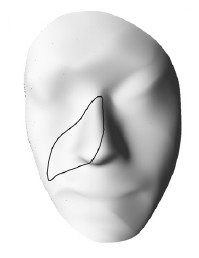Y. J. Yang, S. Cao, J. H. Yong, H. Zhang, J. C. Paul, J. G. Sun.  Approximate computation of curves on B-spline surfaces. Computer-Aided Design, 2008, 40(2):223-234. (SCI: CAIDA5; EI:080611089859,impact factor 1.264)Abstract: Curves on surfaces play an important role in computer-aided geometric design. Because of the considerably high degree of exact curves on surfaces, approximation algorithms are preferred in CAD systems. To approximate the exact curve with a reasonably low degree curve which also lies completely on the B-spline surface, an algorithm is presented in this paper. The Hausdorff distance between the approximate curve and the exact curve is controlled under the user-specified distance tolerance. The approximate curve is approximate continuous. Examples are given to show the performance of our algorithm.Y. J. Yang, J. H. Yong, J. G. Sun. An algorithm for tetrahedral mesh generation based on conforming constrained Delaunay tetrahedralization. Computers & Graphics, 2005, 29(4): 606-615. (SCI: 971CG, EI: 8765028,impact factor 1.0)Abstract: An unstructured tetrahedral mesh generation algorithm for 3D model with constraints is presented. To automatically generate a tetrahedral mesh for model with constraints, an advancing front algorithm is presented based on conforming constrained Delaunay tetrahedralization (CCDT). To reduce the number of visibility tests between vertices with respect to model faces as well as the computation of constrained Delaunay tetrahedra, a sufficient condition for DT (constrained Delaunay tetrahedralization whose simplexes are all Delaunay) existence is presented and utilized coupled to uniform grid and advancing front techniques in our algorithm. The mesh generator is robust and exhibits a linear time complexity for mechanical models with uniform density distribution.Y. J. Yang, J. H. Yong, H. Zhang, J.C. Paul, J.G. Sun. A rational extension of Piegl's method for filling n-sided holes. Computer-Aided Design, 2006, 38(11): 1166-1178. (SCI: 098FP, EI: 063910135166,impact factor 1.234) Abstract: N-sided hole filling plays an important role in vertex blending. To deal with the case that the corner is surrounded by rational surfaces (i.e. NURBS surfaces), an algorithm to fill an n-sided hole with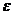-G1 continuous NURBS patches that interpolate the given boundary curves and approximate the given cross-boundary derivatives is presented based on Piegl's method. The NURBS surfaces joining along inner or boundary curves have normal vectors that do not deviate more than the user-specified angular tolerance . The boundaries as well as cross-boundary derivatives all can be NURBS curves. No restrictions are imposed on the number of boundary curves, and the cross-boundary derivatives can be specified independently.Y. J. Yang, Ting-Ting Cui, Cheng-Lei Yang, Xiang-Xu Meng, Wei Zeng. Bezier surfaces with orthogonal iso-parameter curves, GDC 2013, Dalian, China.Abstract: The representation of Bezier surfaces greatly affects the results of rendering and tessellation applications. The orthogonality of iso-parameter curves is one of the most important properties of the surface parameterization in differential geometry. The only rational bilinear surface with orthogonal iso-parameter curves is a rectangle. For Bezier surfaces of degree 2 × 2, we derive the explicit orthogonality condition of their control points, by using which a scheme to construct surfaces with orthogonal iso-parameter curves is then presented. Also the possible extension of the construction scheme for Bezier surfaces of any degree is discussed. Examples are given to show the performance of our algorithm for rendering and tessellation applications.Y. J. Yang, J. H. Yong, H. Zhang, J.C. Paul, J.G. Sun. Optimal parameterizations of Bezier surfaces. 2nd International Symposium on Visual Computing, Reno (Nevada), USA. LNCS 4291, pp. 672-681. 2006. (EI:065010308619)  Abstract: The presentation of Bezier surfaces affects the results of rendering and tessellating applications greatly. To achieve optimal parameterization, we present two reparameterization algorithms using linear Mobius transformations and quadratic transformations, respectively. The quadratic reparameterization algorithm can produce more satisfying results than the Mobius reparameterization algorithm with degree elevation cost. Examples are given to show the performance of our algorithms for rendering and tessellating applications.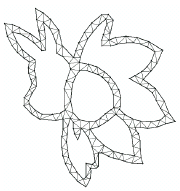Y. J. Yang, H. Zhang, J. H. Yong, J.C. Paul, J.G. Sun. Constrained Delaunay Triangulation Using Delaunay Visibility. 2nd International Symposium on Visual Computing, Reno (Nevada), USA. LNCS 4291, pp. 682-691. 2006. (EI:065010308619) Abstract: An algorithm for constructing constrained Delaunay triangulation (CDT) of a planar straight-line graph (PSLG) is presented. Although the uniform grid method can reduce the time cost of visibility determinations, the time needed to construct the CDT is still long. The algorithm proposed in this paper decreases the number of edges involved in the computation of visibility by replacing traditional visibility with Delaunay visibility. With Delaunay visibility introduced, all strongly Delaunay edges are excluded from the computation of visibility. Furthermore, a sufficient condition for DT (CDT whose triangles are all Delaunay) existence is presented to decrease the times of visibility determinations. The mesh generator is robust and exhibits a linear time complexity for randomly generated PSLGs.